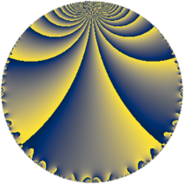# Properties

 Label 15.4.eLevel $15$ Weight $4$ Character orbit 15.e Rep. character $\chi_{15}(2,\cdot)$ Character field $\Q(\zeta_{4})$ Dimension $8$ Newform subspaces $1$ Sturm bound $8$ Trace bound $0$

# Related objects

## Defining parameters

 Level: $$N$$ $$=$$ $$15 = 3 \cdot 5$$ Weight: $$k$$ $$=$$ $$4$$ Character orbit: $$[\chi]$$ $$=$$ 15.e (of order $$4$$ and degree $$2$$) Character conductor: $$\operatorname{cond}(\chi)$$ $$=$$ $$15$$ Character field: $$\Q(i)$$ Newform subspaces: $$1$$ Sturm bound: $$8$$ Trace bound: $$0$$

## Dimensions

The following table gives the dimensions of various subspaces of $$M_{4}(15, [\chi])$$.

Total New Old
Modular forms 16 16 0
Cusp forms 8 8 0
Eisenstein series 8 8 0

## Trace form

 $$8 q - 6 q^{3} - 12 q^{6} - 16 q^{7} + O(q^{10})$$ $$8 q - 6 q^{3} - 12 q^{6} - 16 q^{7} - 100 q^{10} + 132 q^{12} + 68 q^{13} + 90 q^{15} + 284 q^{16} - 240 q^{18} - 492 q^{21} - 500 q^{22} - 220 q^{25} + 702 q^{27} + 508 q^{28} + 660 q^{30} + 616 q^{31} - 240 q^{33} - 804 q^{36} - 1156 q^{37} - 600 q^{40} + 540 q^{42} + 548 q^{43} + 180 q^{45} + 736 q^{46} - 1116 q^{48} - 852 q^{51} + 224 q^{52} + 460 q^{55} + 684 q^{57} + 60 q^{58} + 540 q^{60} + 16 q^{61} + 1428 q^{63} + 2040 q^{66} + 404 q^{67} - 2220 q^{70} - 1800 q^{72} - 2512 q^{73} - 2910 q^{75} - 1488 q^{76} - 360 q^{78} + 288 q^{81} + 2800 q^{82} + 4940 q^{85} - 1680 q^{87} + 2460 q^{88} + 600 q^{90} - 1304 q^{91} + 3408 q^{93} + 4164 q^{96} + 1904 q^{97} + O(q^{100})$$

## Decomposition of $$S_{4}^{\mathrm{new}}(15, [\chi])$$ into newform subspaces

Label Dim $A$ Field CM Traces $q$-expansion
$a_{2}$ $a_{3}$ $a_{5}$ $a_{7}$
15.4.e.a $8$ $0.885$ 8.0.$$\cdots$$.8 None $$0$$ $$-6$$ $$0$$ $$-16$$ $$q-\beta _{3}q^{2}+(-1-\beta _{2}+\beta _{5})q^{3}+(\beta _{2}+\cdots)q^{4}+\cdots$$